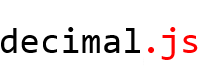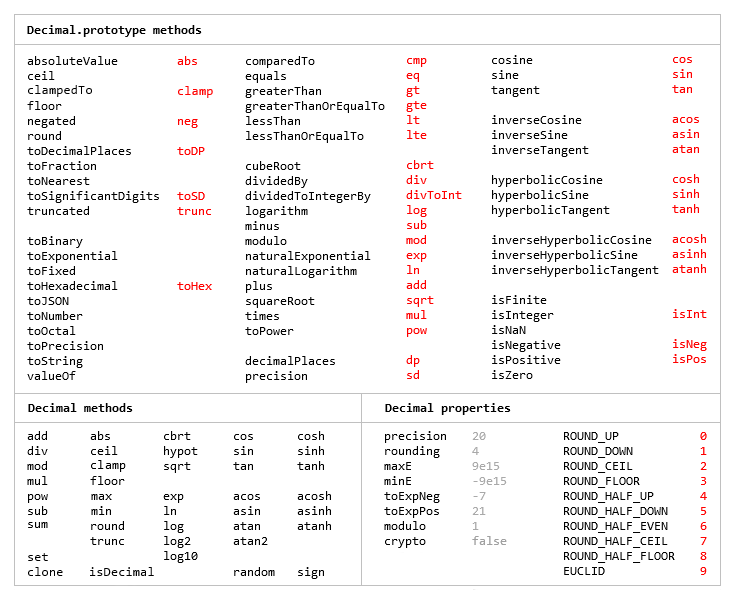# JavaScripting

• ×

## Decimal.js

An arbitrary-precision Decimal type for JavaScript
Filed under  ›

• 🔾70%Overall
• 5,552
• 22.5 days
• 🕩481
• 👥17An arbitrary-precision Decimal type for JavaScript.

## Features

• Integers and floats
• Simple but full-featured API
• Replicates many of the methods of JavaScript's `Number.prototype` and `Math` objects
• Also handles hexadecimal, binary and octal values
• Faster, smaller, and perhaps easier to use than JavaScript versions of Java's BigDecimal
• No dependencies
• Wide platform compatibility: uses JavaScript 1.5 (ECMAScript 3) features only
• Comprehensive documentation and test set
• Used under the hood by math.js
• Includes a TypeScript declaration file: decimal.d.tsThe library is similar to bignumber.js, but here precision is specified in terms of significant digits rather than decimal places, and all calculations are rounded to the precision (similar to Python's decimal module) rather than just those involving division.

This library also adds the trigonometric functions, among others, and supports non-integer powers, which makes it a significantly larger library than bignumber.js and the even smaller big.js.

For a lighter version of this library without the trigonometric functions see decimal.js-light.

The library is the single JavaScript file decimal.js or ES module decimal.mjs.

Browser:

``````<script src='path/to/decimal.js'></script>

<script type="module">
import Decimal from './path/to/decimal.mjs';
...
</script>
``````
``````npm install decimal.js
``````
``````const Decimal = require('decimal.js');

import Decimal from 'decimal.js';

import {Decimal} from 'decimal.js';
``````

## Use

In all examples below, semicolons and `toString` calls are not shown. If a commented-out value is in quotes it means `toString` has been called on the preceding expression.

The library exports a single constructor function, `Decimal`, which expects a single argument that is a number, string or Decimal instance.

``````x = new Decimal(123.4567)
y = new Decimal('123456.7e-3')
z = new Decimal(x)
x.equals(y) && y.equals(z) && x.equals(z)        // true
``````

If using values with more than a few digits, it is recommended to pass strings rather than numbers to avoid a potential loss of precision.

``````// Precision loss from using numeric literals with more than 15 significant digits.
new Decimal(1.0000000000000001)         // '1'
new Decimal(88259496234518.57)          // '88259496234518.56'
new Decimal(99999999999999999999)       // '100000000000000000000'

// Precision loss from using numeric literals outside the range of Number values.
new Decimal(2e+308)                     // 'Infinity'
new Decimal(1e-324)                     // '0'

// Precision loss from the unexpected result of arithmetic with Number values.
new Decimal(0.7 + 0.1)                  // '0.7999999999999999'
``````

As with JavaScript numbers, strings can contain underscores as separators to improve readability.

``````x = new Decimal('2_147_483_647')
``````

String values in binary, hexadecimal or octal notation are also accepted if the appropriate prefix is included.

``````x = new Decimal('0xff.f')            // '255.9375'
y = new Decimal('0b10101100')        // '172'
z = x.plus(y)                        // '427.9375'

z.toBinary()                         // '0b110101011.1111'
z.toBinary(13)                       // '0b1.101010111111p+8'

// Using binary exponential notation to create a Decimal with the value of `Number.MAX_VALUE`.
x = new Decimal('0b1.1111111111111111111111111111111111111111111111111111p+1023')
// '1.7976931348623157081e+308'
``````

Decimal instances are immutable in the sense that they are not changed by their methods.

``````0.3 - 0.1                     // 0.19999999999999998
x = new Decimal(0.3)
x.minus(0.1)                  // '0.2'
x                             // '0.3'
``````

The methods that return a Decimal can be chained.

``````x.dividedBy(y).plus(z).times(9).floor()
x.times('1.23456780123456789e+9').plus(9876.5432321).dividedBy('4444562598.111772').ceil()
``````

Many method names have a shorter alias.

``````x.squareRoot().dividedBy(y).toPower(3).equals(x.sqrt().div(y).pow(3))     // true
x.comparedTo(y.modulo(z).negated() === x.cmp(y.mod(z).neg())              // true
``````

Most of the methods of JavaScript's `Number.prototype` and `Math` objects are replicated.

``````x = new Decimal(255.5)
x.toExponential(5)                       // '2.55500e+2'
x.toFixed(5)                             // '255.50000'
x.toPrecision(5)                         // '255.50'

Decimal.sqrt('6.98372465832e+9823')      // '8.3568682281821340204e+4911'
Decimal.pow(2, 0.0979843)                // '1.0702770511687781839'

// Using `toFixed()` to avoid exponential notation:
x = new Decimal('0.0000001')
x.toString()                             // '1e-7'
x.toFixed()                              // '0.0000001'
``````

And there are `isNaN` and `isFinite` methods, as `NaN` and `Infinity` are valid `Decimal` values.

``````x = new Decimal(NaN)                                           // 'NaN'
y = new Decimal(Infinity)                                      // 'Infinity'
x.isNaN() && !y.isNaN() && !x.isFinite() && !y.isFinite()      // true
``````

There is also a `toFraction` method with an optional maximum denominator argument.

``````z = new Decimal(355)
pi = z.dividedBy(113)        // '3.1415929204'
pi.toFraction()              // [ '7853982301', '2500000000' ]
pi.toFraction(1000)          // [ '355', '113' ]
``````

All calculations are rounded according to the number of significant digits and rounding mode specified by the `precision` and `rounding` properties of the Decimal constructor.

For advanced usage, multiple Decimal constructors can be created, each with their own independent configuration which applies to all Decimal numbers created from it.

``````// Set the precision and rounding of the default Decimal constructor
Decimal.set({ precision: 5, rounding: 4 })

// Create another Decimal constructor, optionally passing in a configuration object
Dec = Decimal.clone({ precision: 9, rounding: 1 })

x = new Decimal(5)
y = new Dec(5)

x.div(3)                           // '1.6667'
y.div(3)                           // '1.66666666'
``````

The value of a Decimal is stored in a floating point format in terms of its digits, exponent and sign, but these properties should be considered read-only.

``````x = new Decimal(-12345.67);
x.d                            // [ 12345, 6700000 ]    digits (base 10000000)
x.e                            // 4                     exponent (base 10)
x.s                            // -1                    sign
``````

For further information see the API reference in the doc directory.

## Test

To run the tests using Node.js from the root directory:

``````npm test
``````

Each separate test module can also be executed individually, for example:

``````node test/modules/toFraction
``````

To run the tests in a browser, open test/test.html.

## Minify

Two minification examples:

Using uglify-js to minify the decimal.js file:

``````npm install uglify-js -g
uglifyjs decimal.js --source-map url=decimal.min.js.map -c -m -o decimal.min.js
``````

Using terser to minify the ES module version, decimal.mjs:

``````npm install terser -g
terser decimal.mjs --source-map url=decimal.min.mjs.map -c -m --toplevel -o decimal.min.mjs
``````
``````import Decimal from './decimal.min.mjs';
``````

## Licence

The MIT Licence (Expat).